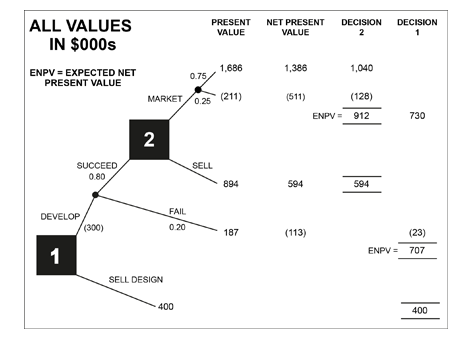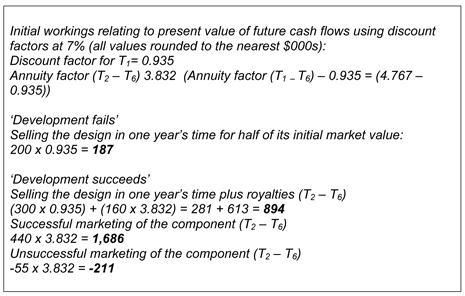# Conditional probability

Management decision making in both financial and performance management involves making choices based upon what may happen in the future. Such future events can be predicted but decision makers can rarely be 100% certain that these future events will actually occur or will occur in the way in which they are forecast.

There are a variety of ways of dealing with such uncertainty in forecast outcomes. In this article, we shall look at how to consider all of the possible outcomes that may arise, together with their associated chances of occurring. This is referred to as a situation where we are evaluating risk, since there is past information or experience which can provide statistical evidence in order to assist in determining the possibility of each event occurring.

### Expected values, decision trees and combined probabilities

A commonly used way of evaluating decisions is via the use of expected values.

An expected value summarises all the different possible outcomes by weighting the possible outcomes by their probabilities and then summing the result.

Problems where one or more decisions have to be taken can become more complex and may require the use of a decision tree, with expected values being used to evaluate each of the decisions.

A decision tree is a diagrammatic representation of a problem, where the decision maker needs to consider the logical sequence of events.

Since one event may depend upon another, we may get situations where event one has a certain probability of occurring and event two, which depends on event one occurring, has another probability of occurring. In such circumstances, we have a situation of combined probabilities

For example, if event one has a 0.6 chance of occurring and subsequent event two a 0.75 chance of occurring, then overall the probability of both events occurring is:

0.6 x 0.75 = 0.45
ie a 45% chance of occurring.

We shall look at such concepts in the following example, which demonstrates how techniques acquired from the Applied Skills exams can be used in the Strategic Professional exams.

### Scenario

Brisport Master Motor Co (Brisport) designs, manufactures and sells a range of components for the motor car industry. The design team has recently designed a new component for inclusion into hybrid cars. The component greatly enhances the battery ‘road time’ and therefore reduces the frequency with which the battery has to be recharged.

The company can either sell the design now, for its initial market value of \$400,000, or attempt to develop the design into a marketable product, which can be supplied to the motor industry. This development would have an initial outlay of \$300,000 now and the component would take one year to be developed. In such a fast moving market, the component is likely to have a market life as a saleable product of just five years after development.

If the company decides to develop the component, the chances of succeeding in developing the design into the marketable product are 80%. If the attempt to develop fails, the design can only be sold, in one year’s time, for half of its earlier market value.

If the attempt to develop the design succeeds the company has a choice of either selling both the design and the rights to sell the developed component, or marketing the component themselves.

Selling the design would yield \$300,000 in one year’s time and \$160,000 in royalty payments for each of the five years thereafter (years 2 to 6).

If the component is marketed by Brisport then there is a 75% probability that the product will be popular and will generate cash inflows of \$440,000 per annum but there is a 25% probability that it will be unpopular and it will generate cash outflows of \$55,000 per annum. Both cash flow figures are also for each of years 2 to 6.

Brisport uses a weighted average cost of capital of 7% to discount its future cash flows.

The management of Brisport Master Motor Co seeks your advice as to their best course of action.

### SolutionThere are two decisions which need to be taken by the company:

1. sell the design or develop it
2. if it is developed, then whether to sell the design and the rights to sell the developed component, or market it themselvesIn order to evaluate decision 1, decision 2 needs to be evaluated first. In other words, the values we use in decision 1 need to be determined by the decision we take in decision 2.

### Decision 2

The net present value (\$000s) on the 75% path is

1,686 – 300 = 1,386.

Taken together with the net present value (\$000s) on the 25% path of

(211) – 300 = (511)

there is an expected net present value of choosing to market the component of (\$000s):

[0.75 x 1,386] + [0.25 x (511)]
=  1,040 – 128
=  912

This is a higher value than the option of selling the design and the rights to sell the developed component for a net present value of \$594,000 (\$894,000 – \$300,000). Therefore, if the development goes ahead, it will be more beneficial to market the product.

Of course, we still need to evaluate decision 1, whether to develop at all. The ‘success’ of the expected present value of \$912,000 in decision 2 has an 80% chance of arising, but there is a 20% chance of the development not succeeding and recouping just half of the initial market value, that being \$187,000 in present value terms, resulting in the company being worse off by \$113,000 in present value terms after taking the development costs into account.

Hence, the expected net present value of the development option of decision 1 can be calculated (\$000s):

= [0.80 x 912] + [0.20 x (113)]
= 730 – 23
= 707

Since this is higher than the option to sell the design at time 0, \$400,000, on an expected value basis, the component should be developed and marketed.

### Attitude to risk

The expected value approach assumes risk neutrality, but not all management decision makers are risk neutral. A risk averse management would, in this scenario, be concerned with the 20% probability of being \$113,000 worse off in present value terms should the development decision go on to fail.

Furthermore, having taken the decision (at node 2) that marketing the component is preferred to selling both the design and developed component there is a further risk of losses, since there is a 25% chance of the component being unpopular leaving the company worse off by \$511,000 in present value terms.

Combined with the 80% probability of the development being successful, there is an overall 20% chance of this \$511,000 loss. This 20% is known as a conditional probability since it depends upon the 80% (0.80) success rate firstly and then depends on the 25% (0.25) unpopularity chance.

Hence,

0.80 x 0.25 = 0.20 ie 20%

For completeness, there is of course a 75% chance of the component being popular if marketed, and hence the overall combined probability of a successful development together with a marketing campaign which results in popularity is:

0.80 x 0.75 = 0.60
ie 60%

### Summary

OutcomeProbabilityNet present value (\$000s)
Development succeeds and component is popular
60%1,386
Development succeeds but component is unpopular
20%(511)
Development fails20%(113)

Therefore, be aware that expected values can lead to a false sense of security. The expected NPV of \$707,000 is an average. In other words, it is the average NPV if the decision is repeated over and over again. But is that useful in this situation? This is a one-off development of a product and therefore only one of the outcomes listed in the table above will actually occur. (This is analogous to tossing a coin once. We know that the outcome will either be a head or a tail, not the expected value of ‘half a head’ or ‘half a tail’). As can be seen above, there is a 40% chance that the NPV will be negative, and that is maybe a risk that the company is not prepared to take.

Furthermore, be aware that the analysis largely depends upon the values of the probabilities prescribed. Often these are subjective estimates made by the decision makers and it would only take relatively small changes in these to alter one of the decisions.

For example, in decision 2, if the probability of successful marketing falls to 55%, then the expected NPV of ‘marketing’ falls to:

[0.55 x 1,386] + [0.45 x (511)]
=  762 – 230
=  532

This is now a lower value than the option of selling the design and the rights to sell the developed component for a net present value of \$594,000.

Such sensitivity analysis can be performed on other variables within the model.

Of course, decision models such as this are only as good as the information used. In reality there would probably be a much wider range of possible outcomes than the discrete outcomes described above. In other words, the problems in examination questions are a simpler version of what is usually found in reality, but nonetheless are very useful techniques of which you should be aware.

Written by a member of the Advanced Financial Management examining team